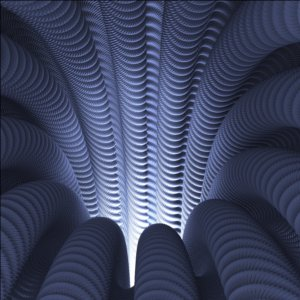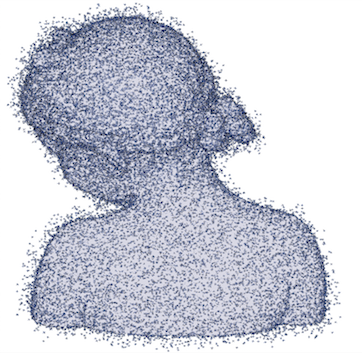Associate professor in applied mathematics, Grenoble, France Université Grenoble Alpes Laboratoire Jean Kuntzmann CVGI TeamCoorganisation of the events:
- French Computational Geometry Days, 9 - 13 March 2020, CIRM, Luminy, France.
- Workshop on Convex Integration and Paradoxical Shapes, August 30 - September 04 2020, Oaxaca, Mexico

New:
Optimal transport: discretization and algorithms
Q. Mérigot, B. Thibert, In Handbook of Numerical Analysis, Vol 22, Geometric PDEs, pp 134-212, 2021.

## Research interests

I am mostly interested in the development of effective calculations in problems having a geometric flavor, which could be refered as applied geometry or numerical geometry. In particular, I have been working in the field of geometric inference and on different nonlinear geometric problems, having connections with various domains such as optimal transport, Monge-Ampère equations, inverse problems in optics, computational geometry or convex integration theory. Below are some of my favorite fields I have contributed to.Numerical optimal transport / inverse problems in optics. I am working on the numerical aspect of optimal transport, in particular in the semi-discrete setting (i.e. when transporting an absolutely continuous measure to a discrete one). I am also investigating applications to inverse problems arising in non-imaging optics. Figure: Simulation with a raytracer of the light projected on a wall after reflexion of an isotropic punctual source light on an optical compound (reflector surface) that we built.Isometric embeddings / Convex Integration theory. I am working with the Hevea Project on the realization of Nash's isometric embeddings based on the implementation of the Convex Integration Theory developped by Gromov in the 70s. I am particularly interested in the simplification of this Convex Integration Theory in order to make it more effective and provide a numerical tool (more) usable in practice to solve some nonlinear partial differential equations. Click here for a concrete application. Figure: inside of an isometric embedding of a flat torus (in the three dimensional space) that reveals a smooth-fractal structureGeometric inference. The aim of geometric inference is to get robust estimations of the topological and geometric properties of a geometric object from an approximation, such as a finite point set. I have in particular been working on the stability of the (Federer) curvature measures, the regularity of the distance functions to compact sets, Voronoi Covariance Measures using distances to measures and have also investigated applications in digital geometry. Figure: Example of a finite point set from which one wants to infer geometric properties of the underlying object.

More details on my publications web page.

## On-going projects

• Hevea: H-principE, Visualisation Et Applications.
• MAGA: Monge Ampère et Géometrie Algorithmique
• CoMeDiC: Convergent Metrics for Digital Calculus

## Contact

Snail Mail
Laboratoire LJK - Bâtiment IMAG
Université Grenoble Alpes
700 Avenue Centrale
38401 Saint-Martin-d'Hères

Accès
Bureau 161 - 1er étage - bâtiment IMAG - Plan

Phone +33 4 57 42 17 84